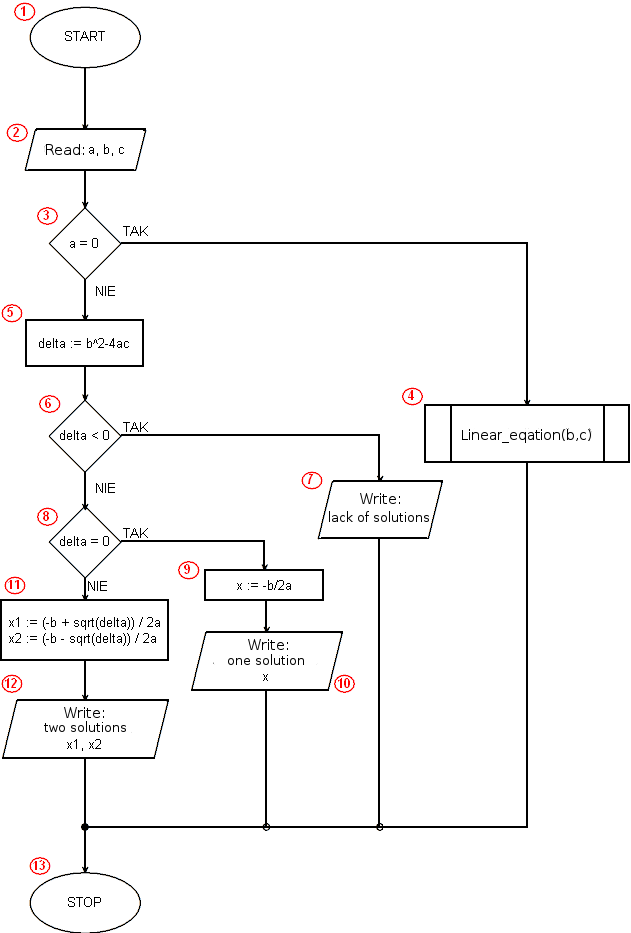# Implementation of the algorithm solving a quadratic equation in Java```import static java.lang.Math.sqrt;
import java.util.Scanner;
public class Square {
public static void main(String[] args) {
double a,b,c;
Scanner key=new Scanner(System.in);
System.out.print("a=");
a=key.nextFloat();
System.out.print("b=");
b=key.nextFloat();
System.out.print("c=");
c=key.nextFloat();
if(a==0)
linear(b,c);
else{
double delta;
delta=b*b-4*a*c;
if(delta<0)
System.out.println("lack of solutions");
else
if(delta==0){
double x;
x=-b/(2*a);
System.out.print("x = ");
System.out.println(x);
}
else{
double x1, x2;
x1=(-b+sqrt(delta))/(2*a);
x2=(-b-sqrt(delta))/(2*a);
System.out.print("x1 = ");
System.out.println(x1);
System.out.print("x2 = ");
System.out.println(x2);
}
}
}
static void linear(double a, double b){
if(a==0)
if(b==0)
System.out.println("identity equation");
else
System.out.println("contrary equation");
else{
double x;
x=-b/a;
System.out.print("linear equation, x = ");
System.out.println(x);
}
}
}```

 Program line Description Block algorithm 1 Our program will use the sqrt function (square root) contained in the Math library. We must import it. - 2 We will also use the Scanner class from the util java library. The Scanner class will be used to load input data in our program. - 3 We begin the definition of the Square class. - 4 We start the definition of the main() function. In java, program execution starts with calling the public main() function contained in the class whose name matches the program name (the program must be saved in the Square.java file and then compiled to the Square.class file). 1 5 We declare variables a, b, c, which we will use in a moment. - 6 Declare and initialize the key variable as a Scanner object. We indicate that the source of text data will be the System.in stream, or keyboard. - 7 - 12 Input/output operations in which the input data is taken - coefficients a, b and c of the equation. At the same time, we display the prompts for the user on the screen. 2 13 A conditional instruction to check if we are dealing with a quadratic equation. If the condition is satisfied (a is equal to 0), our equation is a linear equation. 3 14 The instruction is executed when the condition in the instruction from line 13 has been satisfy (and is equal to 0). We invoke a function that performs the algorithm of solving a linear equation - linear(b, c). 4 15 We start a block of instructions executed when the condition from line 13 is not satisfy, so when a is different from zero, that is, our equation is a quadratic equation. - 16 We declare the variable delta. - 17 We calculate the delta value. 5 18 A conditional statement checking if delta < 0. 6 19 If the condition from line 18 is satisfied (delta < 0), the equation has no solutions. We print a message about the lack of solutions. 7 20 We start the instruction performed when the condition from line 18 has not been satisfy (the delta is greater than or equal to 0). - 21 A conditional statement checking if delta = 0. 8 22 We declare the variable x in which we place the solution (one double solutin - delta = 0). - 23 We calculate and write in the x variable the solution of the equation. 9 24 - 25 We display the calculated result. 10 26 The end of the instructions block for delta 0. - 27 We start the program block executed in the situation when the condition delta = 0 (and earlier delta < 0 and a ≠ 0) was not satisfy. - 28 We declare variables x1 and x2, in which we will place solutions of the equation. - 29 - 30 We calculate solutions and their values we assign to variables x1 and x2. 11 31 - 34 We display the calculated result - two solutions of the quadratic equation. 12 35 - 36 We are finishing the blocks of instructions from lines 27 and 15. - 37 Ending brace main function definition, and therefore the implementation of the entire program. 13 38 - 50 We declare and define the function named linear that implements the algorithm of solving linear equations. 4 51 A brace that ends the definition of the Square class. -